A Quadratic Form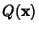is said to be positive definite if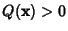for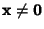. A Real Quadratic Form invariables is positive definite Iff its canonical form is(1)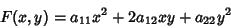(2)

of two Real variables is positive definite if it is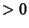for any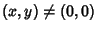, therefore ifand the Discriminant. A Binary Quadratic Form is positive definite if there exist Nonzeroandsuch that(3)

(Le Lionnais 1983).

A Quadratic Formis positive definite Iff every Eigenvalue ofis Positive. A Quadratic Formwitha Hermitian Matrix is positive definite if all the principal minors in the top-left corner ofare Positive, in other words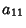(4)(5)(6)